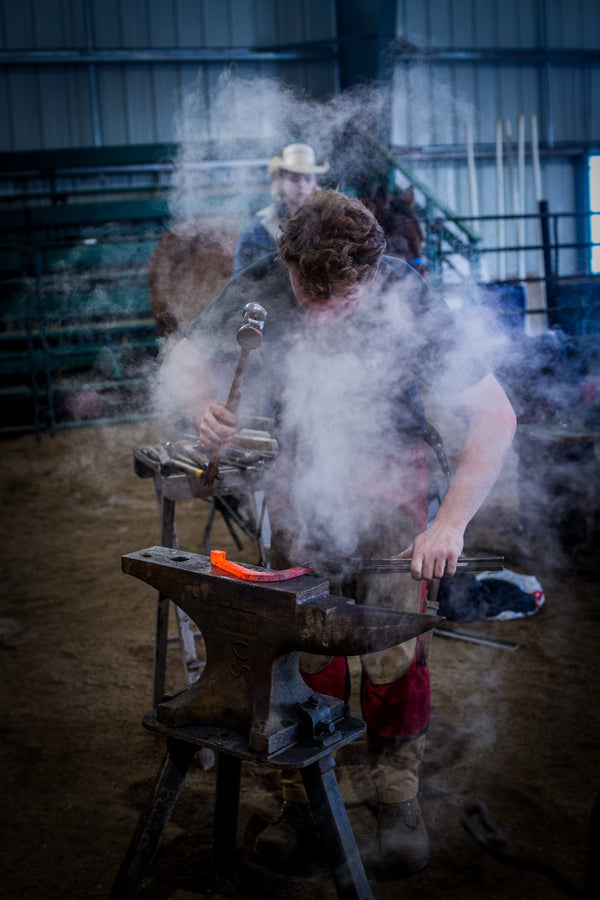0

# Gauging Drill Bit with Handle

Exclusive Pressure-bonded high-speed steel gauging drills. These small diameter precision drills are great for sizing gas orifices, burners, and carburetor jets. The exclusive pressure bonding process provides much closer concentricity than models that are glued together. This enables you to maintain much closer hole size tolerances.

Features:

• 1/4" Hex Handles
• Number drill size stamped on side of the handle for easy identification.

Sizes Available:

• # 53 = 0.0595 Decimal Inches
• # 54 = 0.055 Decimal Inches
• # 55 = 0.052 Decimal Inches
• # 56 = 0.0465 Decimal Inches
• # 60 = 0.04 Decimal Inches
• # 63 = 0.037 Decimal Inches
• # 65 = 0.035 Decimal Inches
• # 67 = 0.032 Decimal Inches
• # 68 = 0.031 Decimal Inches
• # 70 = 0.028 Decimal Inches
• # 72 = 0.025 Decimal Inches
• # 73 = 0.024 Decimal Inches
• # 74 = 0.0225 Decimal Inches

## Subscribe